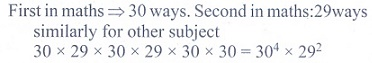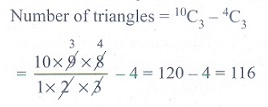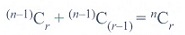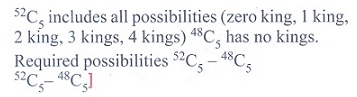Home | | Maths 11th std | Exercise 4.5: Choose the correct or the most suitable answer

# Exercise 4.5: Choose the correct or the most suitable answer

Multiple choice questions with answers / choose the correct answer with answers - Maths Book back 1 mark questions and answers with solution for Exercise Problems - Mathematics : Combinatorics and Mathematical Induction

CHAPTER : Combinatorics and Mathematical Induction

Choose the correct or the most suitable answer

1. The sum of the digits at the 10th place of all numbers formed with the help of 2, 4, 5, 7 taken all at a time is

(1) 432

(2) 108

(3) 36

(4) 18

Solution2. In an examination there are three multiple choice questions and each question has 5 choices .

Number of ways in which a student can fail to get all answer correct is

(1) 125

(2) 124

(3) 64

(4) 63

Solution3. The number of ways in which the following prize be given to a class of 30 boys first and second in mathematics, first and second in physics, first in chemistry and first in English is

(1) 304 x 292

(2) 303 x 293

(3) 302 x 294

(4) 30 x 295.

Solution4. The number of 5 digit numbers all digits of which are odd is

(1) 25

(2) 55

(3) 56

(4) 625.

Solution5. In 3 fingers, the number of ways four rings can be worn is ………..ways.

(1) 43 - 1

(2) 34

(3) 68

(4) 64

Solution

4 rings can be worn in 3 fingers in 3ways

6. If (n+5)P(n+1) = ( 11(n-1) /2 )(n+3)Pn, then the value of n are

(1) 7 and 11

(2) 6 and 7

(3) 2 and 11

(4) 2 and 6.

Solution7. The product of r consecutive positive integers is divisible by

(1) r!

(2) (r - 1)!

(3) (r + 1)!

(4) rr.

Solution

Product of r consecutive positive integers is divisible by r! (theorem)

8. The number of five digit telephone numbers having at least one of their digits repeated is

(1) 90000

(2) 10000

(3) 30240

(4) 69760.

Solution9. If a2-aC2 = a2-a C4 then the value of ’a’ is

(1) 2

(2) 3

(3) 4

(4) 5

Solution10. There are 10 points in a plane and 4 of them are collinear. The number of straight lines joining any two points is

(1) 45

(2) 40

(3) 39

(4) 38.

Solution11. The number of ways in which a host lady invite 8 people for a party of 8 out of 12 people of whom two do not want to attend the party together is

(1) 2 x11C7 +10C8

(2) 11C7 +10C8

(3) 12C8 -10C6

(4) 10C6 + 2!.

Solution12. The number of parallelograms that can be formed from a set of four parallel lines intersecting another set of three parallel lines.

(1) 6

(2) 9

(3) 12

(4) 18

Solution13. Everybody in a room shakes hands with everybody else. The total number of shake hands is 66. The number of persons in the room is

(1) 11

(2) 12

(3) 10

(4) 6

Solution14. Number of sides of a polygon having 44 diagonals is

(1) 4

(2) 4!

(3) 11

(4) 22

Solution15. If 10 lines are drawn in a plane such that no two of them are parallel and no three are concurrent, then the total number of points of intersection are

(1) 45

(2) 40

(3)10!

(4) 210

Solution16. In a plane there are 10 points are there out of which 4 points are collinear, then the number of triangles formed is

(1) 110

(2) 10C3

(3) 120

(4) 116

Solution17. In 2nC3 :n C3 = 11 : 1 then n is

(1) 5

(2) 6

(3)11

(4)7

Solution18. (n-1)Cr +(n-1) C(r-1) is

(1) (n+1)Cr

(2) (n-1)Cr

(3) nCr

(4)nCr-1.

Solution19. The number of ways of choosing 5 cards out of a deck of 52 cards which include at least one king is

(1) 52C5

(2) 48C5

(3)52C5 +48 C5

(4)52C5 -48 C5.

Solution20. The number of rectangles that a chessboard has………..

(1) 81

(2) 99

(3)1296

(4) 6561

Solution21. The number of 10 digit number that can be written by using the digits 2 and 3 is

(1) 10C2 + 9C2

(2) 210

(3)210 - 2

(4) 10!

Solution22. If Pr stands for rPr then the sum of the series 1 + P1 + 2P2 + 3P3 +……….+ nPn is

(1) Pn+1

(2) Pn+1 - 1

(3) Pn-1 + 1

(4)(n+1)P(n-1)

Solution23. The product of first n odd natural numbers equals

(1) 2nCn x nPn

(2) (1/2 )n x2nCn xn Pn

(3) (1/4 )n x 2nCn x 2n Pn

(4)nCn x nPn

Solution24. If nC4, nC5, nC6 are in AP the value of n can be

(1) 14

(2) 11

(3)9

(4)5

Solution25. 1 + 3 + 5 + 7 +……….+ 17 is equal to

(1) 101

(2) 81

(3) 71

(4) 61

SolutionTags : Combinatorics and Mathematical Induction | Mathematics , 11th Mathematics : UNIT 4 : Combinatorics and Mathematical Induction
Study Material, Lecturing Notes, Assignment, Reference, Wiki description explanation, brief detail
11th Mathematics : UNIT 4 : Combinatorics and Mathematical Induction : Exercise 4.5: Choose the correct or the most suitable answer | Combinatorics and Mathematical Induction | Mathematics